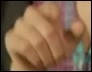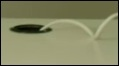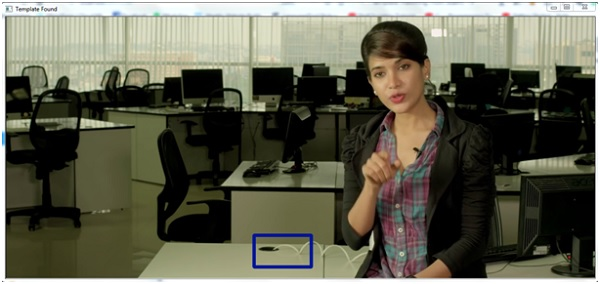# Template matching using OpenCV in Python

The Template matching is a technique, by which a patch or template can be matched from an actual image. This is basically a pattern matching mechanism.

In Python there is OpenCV module. Using openCV, we can easily find the match. So in this problem, the OpenVC template matching techniques are used.

To use the OpenCV functionality, we need to download them using pip.

sudo pip3 install opencv-python


For template matching task, there is an accuracy factor, this factor is known as threshold. As an example, we can say that we can easily create face recognizing scheme using this template matching solution. We can provide some images of eyes or any other parts of faces, then using those images as template, it can easily find the match, but there are different variations in eyes. So if we set the accuracy level to 50%, it will detect better than accuracy level 100%.Generally, the accuracy level is 80% in different cases.

## Steps to match Templates

• Take the actual image and convert it into a gray scale image.

• Take template as gray scale image

• The template slides over the actual image and find the location where accuracy level matches.

• When result is greater than the accuracy level, mark that position as detected.

For the first case the Input image and the template is −

## Main Image## The template## Example code

import cv2
import numpy as np
#open the main image and convert it to gray scale image
gray_image = cv2.cvtColor(main_image, cv2.COLOR_BGR2GRAY)
#open the template as gray scale image
width, height = template.shape[::-1] #get the width and height
#match the template using cv2.matchTemplate
match = cv2.matchTemplate(gray_image, template, cv2.TM_CCOEFF_NORMED)
threshold = 0.8
position = np.where(match >= threshold) #get the location of template in the image
for point in zip(*position[::-1]): #draw the rectangle around the matched template
cv2.rectangle(main_image, point, (point + width, point + height), (0, 204, 153), 0)
cv2.imshow('Template Found', main_image)
cv2.waitKey(0)


## OutputThe above code does not support multi-scaling. So if the size of the template is not same, it will not detect. So in the next part, we will see how we can use the multi-scaling feature to detect templates.

In this approach, the actual image is converted into different sizes, each time it matches the pattern, and finds the largest correlation coefficient to locate the matches.

Here the actual image is same, the template is here −## Example code

import imutils
import cv2
import numpy as np
#Open template and get canny
template = cv2.cvtColor(template, cv2.COLOR_BGR2GRAY)
template = cv2.Canny(template, 10, 25)
(height, width) = template.shape[:2]
#open the main image and convert it to gray scale image
gray_image = cv2.cvtColor(main_image, cv2.COLOR_BGR2GRAY)
temp_found = None
for scale in np.linspace(0.2, 1.0, 20)[::-1]:
#resize the image and store the ratio
resized_img = imutils.resize(gray_image, width = int(gray_image.shape * scale))
ratio = gray_image.shape / float(resized_img.shape)
if resized_img.shape < height or resized_img.shape < width:
break
#Convert to edged image for checking
e = cv2.Canny(resized_img, 10, 25)
match = cv2.matchTemplate(e, template, cv2.TM_CCOEFF)
(_, val_max, _, loc_max) = cv2.minMaxLoc(match)
if temp_found is None or val_max>temp_found:
temp_found = (val_max, loc_max, ratio)
#Get information from temp_found to compute x,y coordinate
(_, loc_max, r) = temp_found
(x_start, y_start) = (int(loc_max), int(loc_max))
(x_end, y_end) = (int((loc_max + width)), int((loc_max + height)))
#Draw rectangle around the template
cv2.rectangle(main_image, (x_start, y_start), (x_end, y_end), (153, 22, 0), 5)
cv2.imshow('Template Found', main_image)
cv2.waitKey(0)


## Output